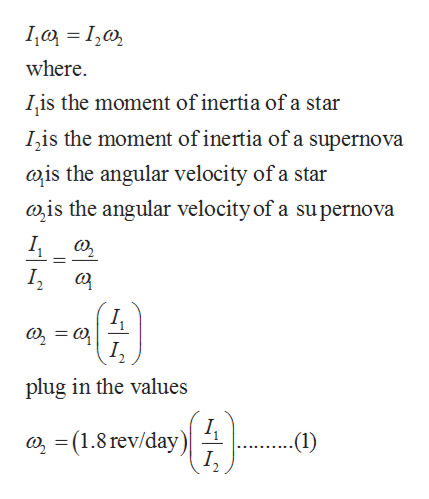# When some stars use up their fuel, they undergo a catastrophic explosion called a supernova. This explosion blows much or all of a star's mass outward, in the form of a rapidly expanding spherical shell. As a simple model of the supernova process, assume that the star is a solid sphere of radius R that is initially rotating at 1.8 revolutions per day. After the star explodes, find the angular velocity, in revolutions per day, of the expanding supernova shell when its radius is 3.9R. Assume that all of the star's original mass is contained in the shell.

Question
401 views

When some stars use up their fuel, they undergo a catastrophic explosion called a supernova. This explosion blows much or all of a star's mass outward, in the form of a rapidly expanding spherical shell. As a simple model of the supernova process, assume that the star is a solid sphere of radius R that is initially rotating at 1.8 revolutions per day. After the star explodes, find the angular velocity, in revolutions per day, of the expanding supernova shell when its radius is 3.9R. Assume that all of the star's original mass is contained in the shell.

check_circle

Step 1

Given:

Radius of a star = R

Radius of supernova shell = 3.9R

Number of revolutions of star per day = 1.8

i.e. angular velocity of star = 1.8 rev/day

Step 2

According to conservation of angular momentum.help_outlineImage Transcriptionclosewhere. Iis the moment of inertia of a star Iis the moment of inertia of a supernova ais the angular velocity of a star is the angular velocity of a supernova I, plug in the values a (1.8 rev/day) I, -(1) fullscreen
Step 3

The moment of inertia of a solid s...

### Want to see the full answer?

See Solution

#### Want to see this answer and more?

Solutions are written by subject experts who are available 24/7. Questions are typically answered within 1 hour.*

See Solution
*Response times may vary by subject and question.
Tagged in

### Rotational Mechanics